刨死你系列——HashMap剖析(基于jdk1.8)

HashMap:
Hash表是一个数组+链表的结构，这种结构能够保证在遍历与增删的过程中，如果不产生hash碰撞，仅需一次定位就可完成，时间复杂度能保证在O(1)。  在jdk1.7中，只是单纯的数组+链表的结构，但是如果散列表中的hash碰撞过多时，会造成效率的降低，所以在JKD1.8中对这种情况进行了控制，当一个hash值上的链表长度大于8时，该节点上的数据就不再以链表进行存储，而是转成了一个红黑树。

static final class TreeNode<K,V> extends LinkedHashMap.Entry<K,V> {
TreeNode<K,V> parent;  // red-black tree links
TreeNode<K,V> left;
TreeNode<K,V> right;
TreeNode<K,V> prev;    // needed to unlink next upon deletion
boolean red;
}

hash碰撞：
hash是指，两个元素通过hash函数计算出的值是一样的，是同一个存储地址。当后面的元素要插入到这个地址时，发现已经被占用了，这时候就产生了hash冲突

hash冲突的解决方法：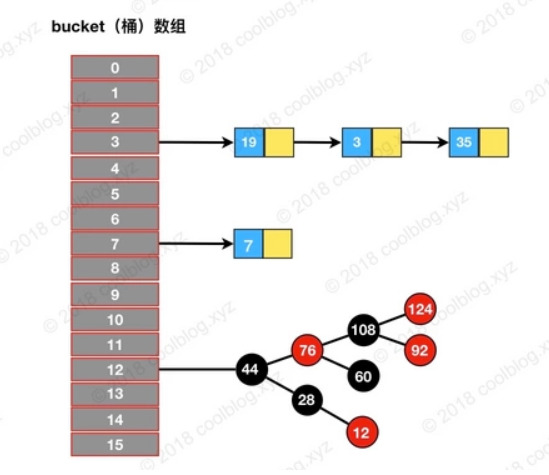Node是HhaspMap中的一个静态内部类 ：

static class Node<K,V> implements Map.Entry<K,V> {
final int hash;
final K key;
V value;
Node<K,V> next;

Node(int hash, K key, V value, Node<K,V> next) {
this.hash = hash;
this.key = key;
this.value = value;
this.next = next;
}

public final K getKey()        { return key; }
public final V getValue()      { return value; }
public final String toString() { return key + "=" + value; }

//hashCode等其他代码
}

//默认初始容量为16，0000 0001 左移4位 0001 0000为16，主干数组的初始容量为16，而且这个数组
//必须是2的倍数(后面说为什么是2的倍数)
static final int DEFAULT_INITIAL_CAPACITY = 1 << 4; // aka 16

//最大容量为int的最大值除2
static final int MAXIMUM_CAPACITY = 1 << 30;

//默认加载因子为0.75
static final float DEFAULT_LOAD_FACTOR = 0.75f;

//阈值，如果主干数组上的链表的长度大于8，链表转化为红黑树
static final int TREEIFY_THRESHOLD = 8;

//hash表扩容后，如果发现某一个红黑树的长度小于6，则会重新退化为链表
static final int UNTREEIFY_THRESHOLD = 6;

//当hashmap容量大于64时，链表才能转成红黑树
static final int MIN_TREEIFY_CAPACITY = 64;

//临界值=主干数组容量*负载因子
int threshold；

HashMap的构造方法：

public HashMap(int initialCapacity, float loadFactor) {
//初始容量小于0，抛出非法数据异常
if (initialCapacity < 0)
throw new IllegalArgumentException("Illegal initial capacity: " +
initialCapacity);
//初始容量最大为MAXIMUM_CAPACITY
if (initialCapacity > MAXIMUM_CAPACITY)
initialCapacity = MAXIMUM_CAPACITY;
//负载因子必须大于0，并且是合法数字
throw new IllegalArgumentException("Illegal load factor: " +

//将初始容量转成2次幂
this.threshold = tableSizeFor(initialCapacity);
}

//tableSizeFor的作用就是，如果传入A，当A大于0，小于定义的最大容量时，
//  如果A是2次幂则返回A，否则将A转化为一个比A大且差距最小的2次幂。
//例如传入7返回8，传入8返回8，传入9返回16
static final int tableSizeFor(int cap) {
int n = cap - 1;
n |= n >>> 1;
n |= n >>> 2;
n |= n >>> 4;
n |= n >>> 8;
n |= n >>> 16;
return (n < 0) ? 1 : (n >= MAXIMUM_CAPACITY) ? MAXIMUM_CAPACITY : n + 1;
}

//调用上面的构造方法，自定义初始容量，负载因子为默认的0.75
public HashMap(int initialCapacity) {
}

//默认构造方法，负载因子为0.75，初始容量为DEFAULT_INITIAL_CAPACITY=16，初始容量在第一次put时才会初始化
public HashMap() {
this.loadFactor = DEFAULT_LOAD_FACTOR; // all other fields defaulted
}

//传入一个MAP集合的构造方法
public HashMap(Map<? extends K, ? extends V> m) {
putMapEntries(m, false);
}

HashMap的put()方法

put 方法的源码分析是本篇的一个重点，因为通过该方法我们可以窥探到 HashMap 在内部是如何进行数据存储的，所谓的数组+链表+红黑树的存储结构是如何形成的，又是在何种情况下将链表转换成红黑树来优化性能的。带着一系列的疑问，我们看这个 put 方法：

public V put(K key, V value) {
return putVal(hash(key), key, value, false, true);
}

static final int hash(Object key) {
int h;
return (key == null) ? 0 : (h = key.hashCode()) ^ (h >>> 16);
}

//onlyIfAbsent是true的话，不要改变现有的值
//evict为true的话，表处于创建模式
final V putVal(int hash, K key, V value, boolean onlyIfAbsent,
boolean evict) {
Node<K,V>[] tab; Node<K,V> p; int n, i;
//如果主干上的table为空，长度为0，调用resize方法，调整table的长度（resize方法在下图中）
if ((tab = table) == null || (n = tab.length) == 0)
/* 这里调用resize，其实就是第一次put时，对数组进行初始化。
如果是默认构造方法会执行resize中的这几句话：
newCap = DEFAULT_INITIAL_CAPACITY;  新的容量等于默认值16
newThr = (int)(DEFAULT_LOAD_FACTOR * DEFAULT_INITIAL_CAPACITY);
threshold = newThr;   临界值等于16*0.75
Node<K,V>[] newTab = (Node<K,V>[])new Node[newCap];
table = newTab; 将新的node数组赋值给table，然后return newTab

如果是自定义的构造方法则会执行resize中的：
int oldThr = threshold;
newCap = oldThr;   新的容量等于threshold，这里的threshold都是2的倍数，原因在
于传入的数都经过tableSizeFor方法，返回了一个新值，上面解释过
float ft = (float)newCap * loadFactor;
newThr = (newCap < MAXIMUM_CAPACITY && ft < (float)MAXIMUM_CAPACITY ?
(int)ft : Integer.MAX_VALUE);
threshold = newThr; 新的临界值等于 (int)(新的容量*负载因子)
Node<K,V>[] newTab = (Node<K,V>[])new Node[newCap];
table = newTab; return newTab;
*/
n = (tab = resize()).length;  //将调用resize后构造的数组的长度赋值给n
if ((p = tab[i = (n - 1) & hash]) == null) //将数组长度与计算得到的hash值比较
tab[i] = newNode(hash, key, value, null);//位置为空，将i位置上赋值一个node对象
else {  //位置不为空
Node<K,V> e; K k;
if (p.hash == hash &&  // 如果这个位置的old节点与new节点的key完全相同
((k = p.key) == key || (key != null && key.equals(k))))
e = p;             // 则e=p
else if (p instanceof TreeNode) // 如果p已经是树节点的一个实例，既这里已经是树了
e = ((TreeNode<K,V>)p).putTreeVal(this, tab, hash, key, value);
else {  //p与新节点既不完全相同，p也不是treenode的实例
for (int binCount = 0; ; ++binCount) {  //一个死循环
if ((e = p.next) == null) {   //e=p.next,如果p的next指向为null
p.next = newNode(hash, key, value, null);  //指向一个新的节点
if (binCount >= TREEIFY_THRESHOLD - 1) // 如果链表长度大于等于8
treeifyBin(tab, hash);  //将链表转为红黑树
break;
}
if (e.hash == hash &&  //如果遍历过程中链表中的元素与新添加的元素完全相同，则跳出循环
((k = e.key) == key || (key != null && key.equals(k))))
break;
p = e; //将p中的next赋值给p,即将链表中的下一个node赋值给p，
//继续循环遍历链表中的元素
}
}
if (e != null) { //这个判断中代码作用为：如果添加的元素产生了hash冲突，那么调用
//put方法时，会将他在链表中他的上一个元素的值返回
V oldValue = e.value;
if (!onlyIfAbsent || oldValue == null)  //判断条件成立的话，将oldvalue替换
//为newvalue，返回oldvalue；不成立则不替换，然后返回oldvalue
e.value = value;
afterNodeAccess(e);  //这个方法在后面说
return oldValue;
}
}
++modCount;  //记录修改次数
if (++size > threshold)   //如果元素数量大于临界值，则进行扩容
resize();   //下面说
afterNodeInsertion(evict);
return null;
}

//将桶内所有的 链表节点 替换成 红黑树节点
final void treeifyBin(Node<K,V>[] tab, int hash) {
int n, index; Node<K,V> e;
//如果当前哈希表为空，或者哈希表中元素的个数小于 进行树形化的阈值(默认为 64)，就去新建/扩容
if (tab == null || (n = tab.length) < MIN_TREEIFY_CAPACITY)
resize();
else if ((e = tab[index = (n - 1) & hash]) != null) {
//如果哈希表中的元素个数超过了 树形化阈值，进行树形化
// e 是哈希表中指定位置桶里的链表节点，从第一个开始
TreeNode<K,V> hd = null, tl = null; //红黑树的头、尾节点
do {
//新建一个树形节点，内容和当前链表节点 e 一致
TreeNode<K,V> p = replacementTreeNode(e, null);
if (tl == null) //确定树头节点
hd = p;
else {
p.prev = tl;
tl.next = p;
}
tl = p;
} while ((e = e.next) != null);
//让桶的第一个元素指向新建的红黑树头结点，以后这个桶里的元素就是红黑树而不是链表了
if ((tab[index] = hd) != null)
hd.treeify(tab);
}
}
TreeNode<K,V> replacementTreeNode(Node<K,V> p, Node<K,V> next) {
return new TreeNode<>(p.hash, p.key, p.value, next);
}

//如果 table 还未被初始化，那么初始化它
if ((tab = table) == null || (n = tab.length) == 0)
n = (tab = resize()).length;

resize()扩容机制，单元素如何散列到新的数组中，链表中的元素如何散列到新的数组中，红黑树中的元素如何散列到新的数组中？

//上图中说了默认构造方法与自定义构造方法第一次执行resize的过程，这里再说一下扩容的过程
final Node<K,V>[] resize() {
Node<K,V>[] oldTab = table;
int oldCap = (oldTab == null) ? 0 : oldTab.length;
int oldThr = threshold;
int newCap, newThr = 0;
if (oldCap > 0) {  //扩容肯定执行这个分支
if (oldCap >= MAXIMUM_CAPACITY) {   //当容量超过最大值时，临界值设置为int最大值
threshold = Integer.MAX_VALUE;
return oldTab;
}
else if ((newCap = oldCap << 1) < MAXIMUM_CAPACITY &&
oldCap >= DEFAULT_INITIAL_CAPACITY) //扩容容量为2倍，临界值为2倍
newThr = oldThr << 1;
}
else if (oldThr > 0) // 不执行
newCap = oldThr;
else {                // 不执行
newCap = DEFAULT_INITIAL_CAPACITY;
newThr = (int)(DEFAULT_LOAD_FACTOR * DEFAULT_INITIAL_CAPACITY);
}
if (newThr == 0) {  // 不执行
float ft = (float)newCap * loadFactor;
newThr = (newCap < MAXIMUM_CAPACITY && ft < (float)MAXIMUM_CAPACITY ?
(int)ft : Integer.MAX_VALUE);
}
threshold = newThr;    //将新的临界值赋值赋值给threshold
@SuppressWarnings({"rawtypes","unchecked"})
Node<K,V>[] newTab = (Node<K,V>[])new Node[newCap];
table = newTab;   //新的数组赋值给table

//扩容后，重新计算元素新的位置
if (oldTab != null) {   //原数组
for (int j = 0; j < oldCap; ++j) {   //通过原容量遍历原数组
Node<K,V> e;
if ((e = oldTab[j]) != null) {   //判断node是否为空，将j位置上的节点
//保存到e,然后将oldTab置为空，这里为什么要把他置为空呢，置为空有什么好处吗？？
//难道是吧oldTab变为一个空数组，便于垃圾回收？？ 这里不是很清楚
oldTab[j] = null;
if (e.next == null)          //判断node上是否有链表
newTab[e.hash & (newCap - 1)] = e; //无链表，确定元素存放位置，
//扩容前的元素地址为 (oldCap - 1) & e.hash ,所以这里的新的地址只有两种可能，一是地址不变，
//二是变为 老位置+oldCap
else if (e instanceof TreeNode)
((TreeNode<K,V>)e).split(this, newTab, j, oldCap);
else { // preserve order
Node<K,V> loHead = null, loTail = null;
Node<K,V> hiHead = null, hiTail = null;
Node<K,V> next;

举个栗子来说一下上面的两种情况：
设：oldCap=16 二进制为：0001 0000
oldCap-1=15 二进制为：0000 1111
e1.hash=10 二进制为：0000 1010
e2.hash=26 二进制为：0101 1010
e1在扩容前的位置为：e1.hash & oldCap-1  结果为：0000 1010
e2在扩容前的位置为：e2.hash & oldCap-1  结果为：0000 1010
结果相同，所以e1和e2在扩容前在同一个链表上，这是扩容之前的状态。

现在扩容后，需要重新计算元素的位置，在扩容前的链表中计算地址的方式为e.hash & oldCap-1
那么在扩容后应该也这么计算呀，扩容后的容量为oldCap*2=32 0010 0000 newCap=32，新的计算
方式应该为
e1.hash & newCap-1
即：0000 1010 & 0001 1111
结果为0000 1010与扩容前的位置完全一样。
e2.hash & newCap-1
即：0101 1010 & 0001 1111
结果为0001 1010,为扩容前位置+oldCap。
而这里却没有e.hash & newCap-1 而是 e.hash & oldCap，其实这两个是等效的，都是判断倒数第五位
是0，还是1。如果是0，则位置不变，是1则位置改变为扩容前位置+oldCap。
再来分析下loTail loHead这两个的执行过程（假设(e.hash & oldCap) == 0成立）：
第一次执行：
e指向oldTab[j]所指向的node对象，即e指向该位置上链表的第一个元素
最后，在判断条件e指向next，就是指向oldTab链表中的第二个元素
第二次执行：
lotail不为null，所以lotail.next指向e，这里其实是lotail指向的node对象的next指向e，
的node变成了一个长度为2的链表。然后lotail=e也就是指向了链表中第二个元素的地址。
第三次执行：
......
历完链表。   这是(e.hash & oldCap) == 0成立的时候。
(e.hash & oldCap) == 0不成立的情况也相同，其实就是把oldCap遍历成两个新的链表，
newTab[j+oldCap]上面去
*/
do {
next = e.next;
if ((e.hash & oldCap) == 0) {
if (loTail == null)
else
loTail.next = e;
loTail = e;
}
else {
if (hiTail == null)
else
hiTail.next = e;
hiTail = e;
}
} while ((e = next) != null);
if (loTail != null) {
loTail.next = null;   //尾节点的next设置为空
}
if (hiTail != null) {
hiTail.next = null;   //尾节点的next设置为空
newTab[j + oldCap] = hiHead;
}
}
}
}
}
return newTab;
}

/**
* Transfers all entries from current table to newTable.
*/
void transfer(Entry[] newTable) {
Entry[] src = table;
int newCapacity = newTable.length;
for (int j = 0; j < src.length; j++) {
Entry<K,V> e = src[j];
if (e != null) {
src[j] null;
do {
// B线程执行到这里之后就暂停了
Entry<K,V> next = e.next;
int i = indexFor(e.hash, newCapacity);
e.next = newTable[i];
newTable[i] = e;
e = next;
} while (e != null);
}
}
}

1）假设我们有两个线程。我用红色和浅蓝色标注了一下。我们再回头看一下我们的 transfer代码中的这个细节：

do {

Entry<K,V> next = e.next; // <--假设线程一执行到这里就被调度挂起了

int i = indexFor(e.hash, newCapacity);

e.next = newTable[i];

newTable[i] = e;

e = next;

} while (e != null);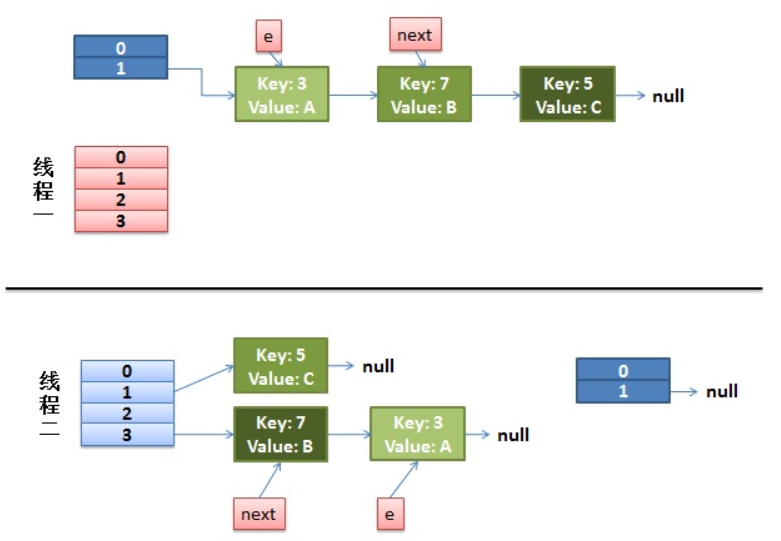2）线程一被调度回来执行。

• 先是执行 newTalbe[i] = e;
• 然后是e = next，导致了e指向了key(7)，
• 而下一次循环的next = e.next导致了next指向了key(3)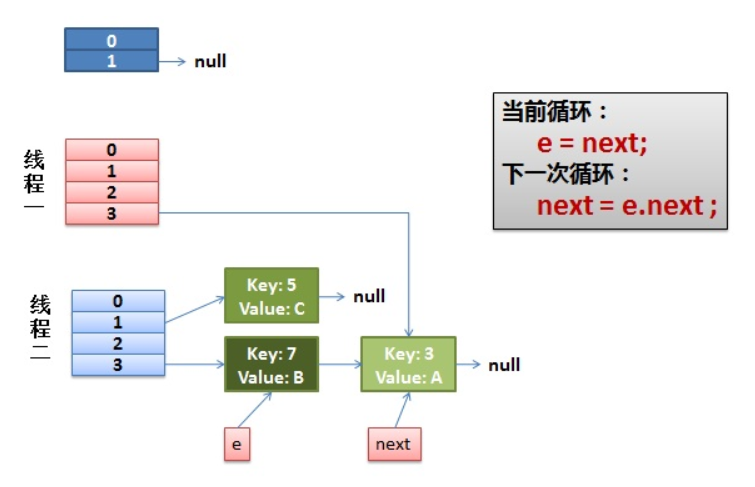3）一切安好。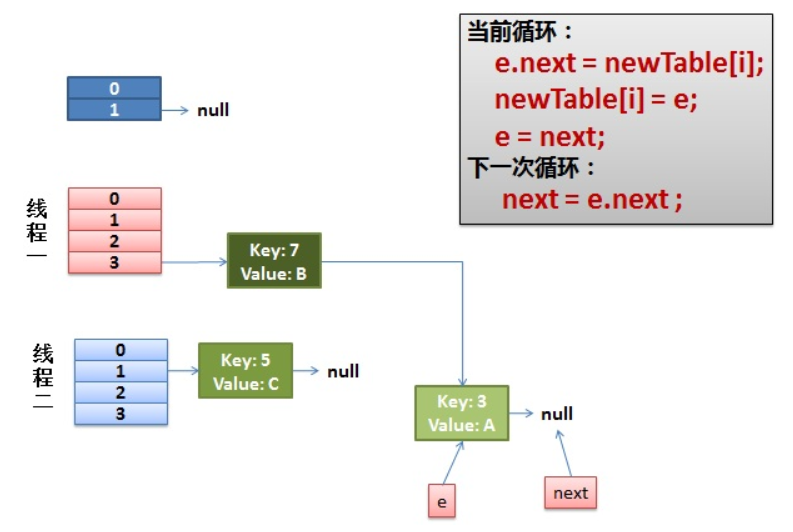4）环形链接出现。

e.next = newTable[i] 导致  key(3).next 指向了 key(7)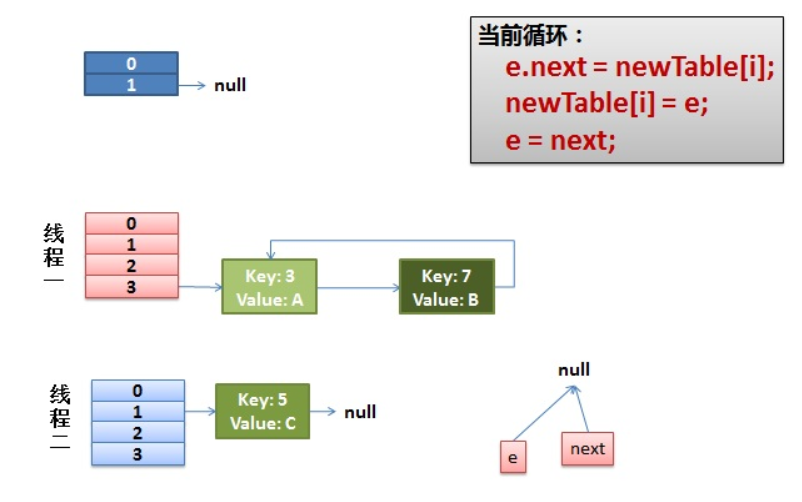于是，当我们的线程一调用到，HashTable.get(11)时，悲剧就出现了——Infinite Loop。

HashMap的get()方法

public V get(Object key) {
Node<K,V> e;
//直接调用了getNode()
return (e = getNode(hash(key), key)) == null ? null : e.value;
}
final Node<K,V> getNode(int hash, Object key) {
Node<K,V>[] tab; Node<K,V> first, e; int n; K k;
//先判断数组是否为空，长度是否大于0，那个node节点是否存在
if ((tab = table) != null && (n = tab.length) > 0 &&
(first = tab[(n - 1) & hash]) != null) {
//如果找到，直接返回
if (first.hash == hash && // always check first node
((k = first.key) == key || (key != null && key.equals(k))))
return first;
if ((e = first.next) != null) {
//如果是红黑树，去红黑树找
if (first instanceof TreeNode)
return ((TreeNode<K,V>)first).getTreeNode(hash, key);
//链表找
do {
if (e.hash == hash &&
((k = e.key) == key || (key != null && key.equals(k))))
return e;
} while ((e = e.next) != null);
}
}
return null;
}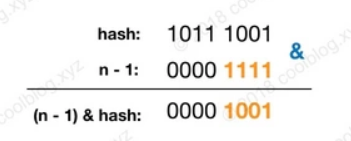在上面源码中，除了查找相关逻辑，还有一个计算 hash 的方法。这个方法源码如下：

/**
* 计算键的 hash 值
*/
static final int hash(Object key) {
int h;
return (key == null) ? 0 : (h = key.hashCode()) ^ (h >>> 16);
}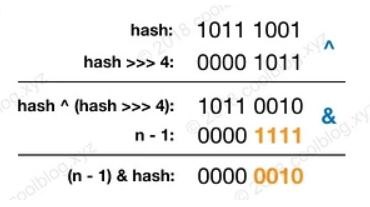https://www.jianshu.com/p/dde9b12343c1

https://www.cnblogs.com/wenbochang/archive/2018/02/22/8458756.html

https://segmentfault.com/a/1190000012926722

https://blog.csdn.net/pange1991/article/details/82377980

posted @ 2019-09-06 09:30 菜鸟小于 阅读(...) 评论(...) 编辑 收藏